Function Repository Resource:

# ProductRule

Represent the derivative of a product, explicitly displaying the terms

Contributed by: Wolfram|Alpha Math Team
 ResourceFunction["ProductRule"][f,x] computes the derivative of the product f with respect to variable x, returning an Inactive sum of product rule terms.

## Details

ResourceFunction["ProductRule"] accepts the following options:
 "PrintRule" False print a statement of the product rule "PrintNapkin" False print the side "napkin" work needed for computing components of the product rule “InactiveInnerDerivatives" False keep the derivatives of the components inactivated

## Examples

### Basic Examples (1)

See how to apply the product rule to compute the derivative of sin(x) * x2 with respect to x:

 In:=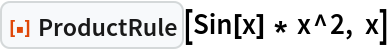Out=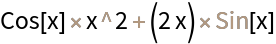### Scope (2)

If there is no explicit product in the first argument of ProductRule, one of the factors is taken to be 1:

 In:=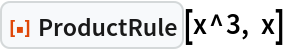Out=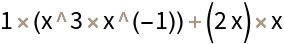If there is a product with multiple factors in the first argument of ProductRule, the factors are split into two separate products:

 In:=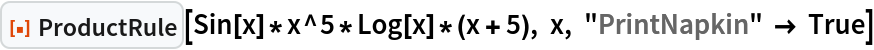Out=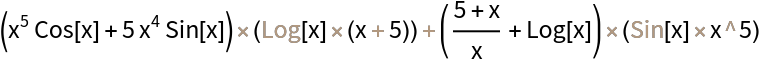### Options (3)

#### InactiveInnerDerivatives (1)

Setting "InactiveInnerDerivatives" to True inactivates the derivatives of the factors within the structure of the product rule:

 In:=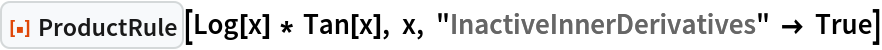Out=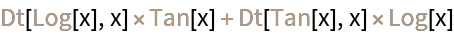#### PrintNapkin (1)

Setting "PrintNapkin" to True prints a grid with the side "napkin" work needed to apply the product rule (i.e., identifying and computing the derivatives of each factor):

 In:=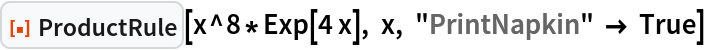Out=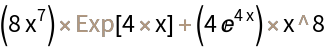#### PrintRule (1)

Setting "PrintRule" to True prints a general statement of the product rule for derivatives:

 In:=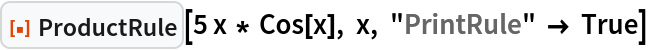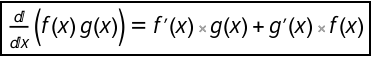Out=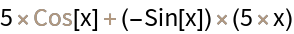## Publisher

Wolfram|Alpha Math Team

## Version History

• 2.0.0 – 23 March 2023
• 1.0.0 – 30 December 2022

## Author Notes

To view the full source code for ProductRule, evaluate the following: